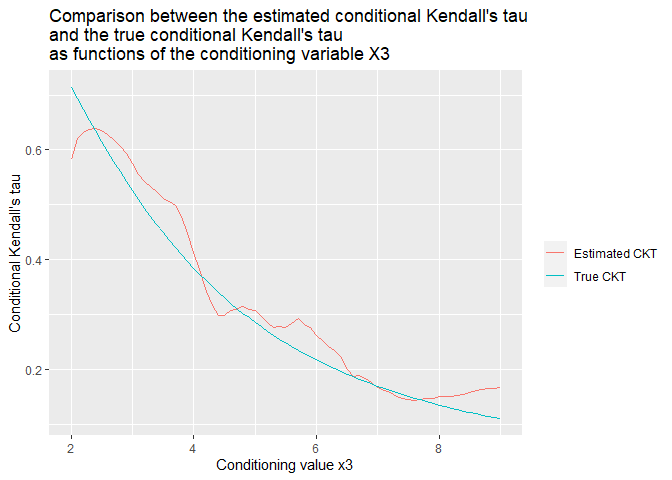# Simulation and estimation from conditional copula models

library(CondCopulas)
library(VineCopula)
library(ggplot2)
set.seed(1)

# Simulation of the data

We simulate a 3 dimensional dataset with one conditioning variable $$X_3$$, following a normal distribution. Conditionally to $$X_3 = x_3$$, we simulate the two variables $$X_1$$ and $$X_2$$ as follows :

• the conditional marginal law of $$X_1$$ given $$X_3 = x_3$$ is normal with mean $$\sin(x_3)$$ and standard deviation $$|x_3|$$ ;

• the conditional marginal law of $$X_2$$ given $$X_3 = x_3$$ is exponential with rate $$\exp(x_3)$$ ;

• the conditional copula between $$X_1$$ and $$X_2$$ given $$X_3 = x_3$$ belongs to the Clayton family with a conditional Kendall’s tau equal to $$1/(1 + 0.1 x_3^2)$$.

Note that this is a conditional model where both the conditional margins and the conditional copula depends on $$X_3$$. We simulate $$n = 2 000$$ observations $$(X_{i,1}, X_{i,2}, X_{i,3})$$ from this model.

n = 2000

meanX3 = 5
sdX3 = 2
X3 = rnorm(n = n, mean = meanX3, sd = sdX3)

# Computation of conditional parameters
meanX1 = sin(X3)
sdX1 = abs(X3)/2

rateX2 = exp(X3/4)

ckt12_3_fun = function(x){return(1 / (1 + 0.1*(x)^2))}
ckt12_3 = ckt12_3_fun(X3)

ggplot() + geom_line(aes(X3, ckt12_3)) +
ggtitle("Conditional Kendall's tau between X1 and X2 conditionally to X3 = x3") +
xlab("Value of x3") + ylab("Conditional Kendall's tau given X3 = x3")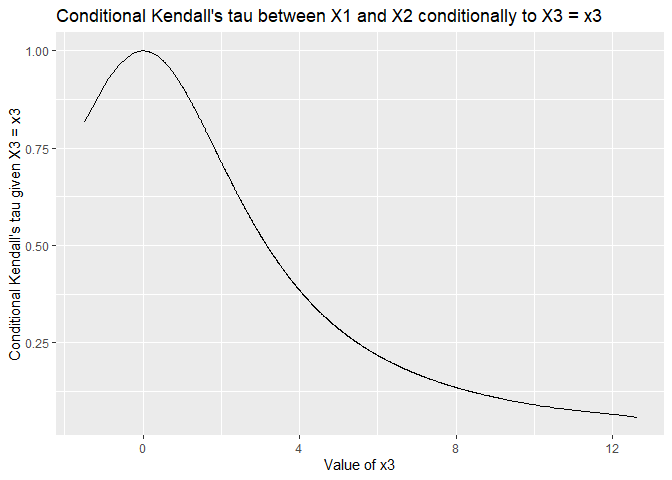copFamily12_3 = 3

# Simulation of X1 and X2
X1 = rep(NA, n)
X2 = rep(NA, n)

for (i in 1:n) {
simCopula = BiCopSim(N=1 , family = copFamily12_3, par = BiCopTau2Par(copFamily12_3 , ckt12_3[i] ))
X1[i] = qnorm(simCopula, mean = meanX1[i], sd = sdX1[i])
X2[i] = qexp(simCopula, rate = rateX2[i])
}

# Estimation of the conditional margins

To have a more visual perspective, we first look at the scatterplots of $$(X_1, X_3)$$ and $$(X_2, X_3)$$ to examine the influence of the conditioning variables $$X_3$$ on the two margins.

ggplot() + geom_point(aes(X3, X1))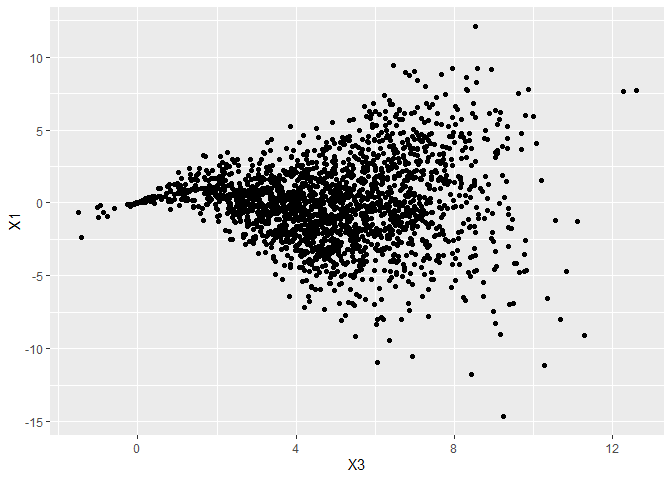ggplot() + geom_point(aes(X3, X2))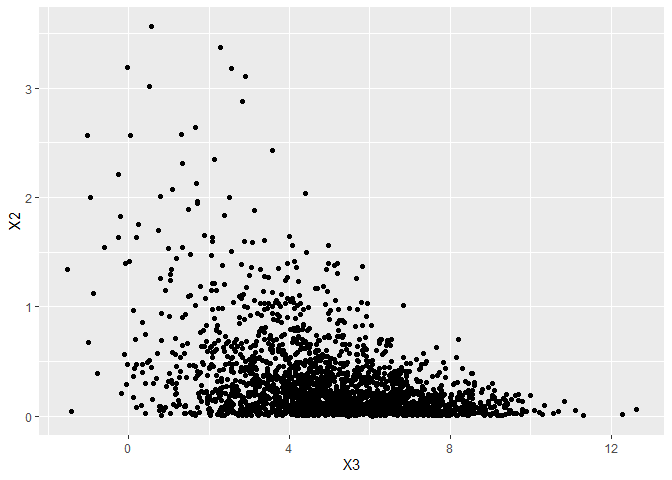We can easily recognize the laws that we simulated from in the previous part. To confirm this, we can estimate the conditional quantiles.

newX3 = c(1,4,8,11)
matrixK = computeKernelMatrix(observedX = X3, newX = newX3, kernel = "Gaussian", h = 0.5)

gridXi = seq(0, 1, by = 0.01)
matrixCondQuantilesX1 = estimateCondQuantiles(observedX1 = X1, probsX1 = gridXi, matrixK)
matrixCondQuantilesX2 = estimateCondQuantiles(observedX1 = X2, probsX1 = gridXi, matrixK)
dataCondQuantiles = data.frame(
pX = gridXi,
qX1 = as.numeric(matrixCondQuantilesX1),
qX2 = as.numeric(matrixCondQuantilesX2),
valX3 = rep(newX3, each = length(gridXi)))

ggplot(dataCondQuantiles, aes(x = pX, y = qX1, color = valX3, group = as.factor(valX3))) + geom_line() +
ggtitle("Conditional quantiles of X1 given X3 = x3") +
xlab("Level of the conditional quantile") +
ylab("Value of the conditional quantile") + scale_color_continuous(name = "Conditioning\nvalue x3")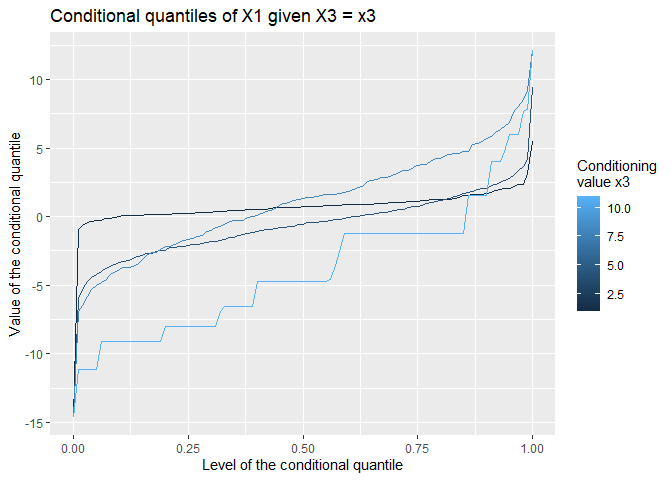ggplot(dataCondQuantiles, aes(x = pX, y = qX2, color = valX3, group = as.factor(valX3))) + geom_line() +
ggtitle("Conditional quantiles of X2 given X3 = x3") +
xlab("Level of the conditional quantile") +
ylab("Value of the conditional quantile") + scale_color_continuous(name = "Conditioning\nvalue x3")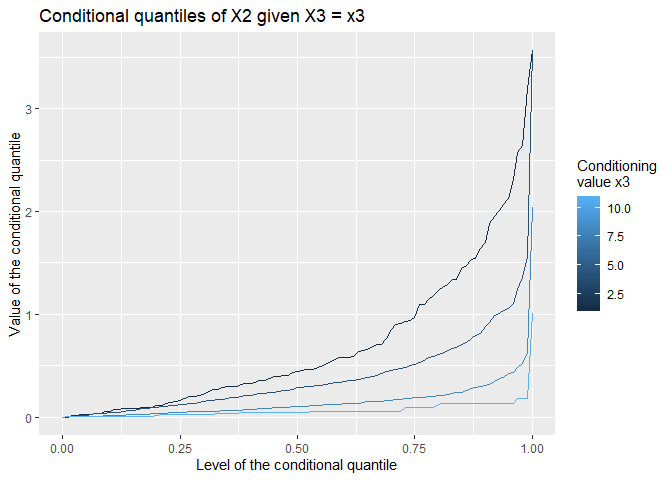# Visualization of the conditional dependence

First, we do a scatterplot of $$(X_1, X_2)$$ where the color of the points is determined by the value of $$X_3$$.

ggplot() + geom_point(aes(x=X1, y=X2, color = X3)) +
ggtitle("Scatterplot of (X1, X2) colored by X3")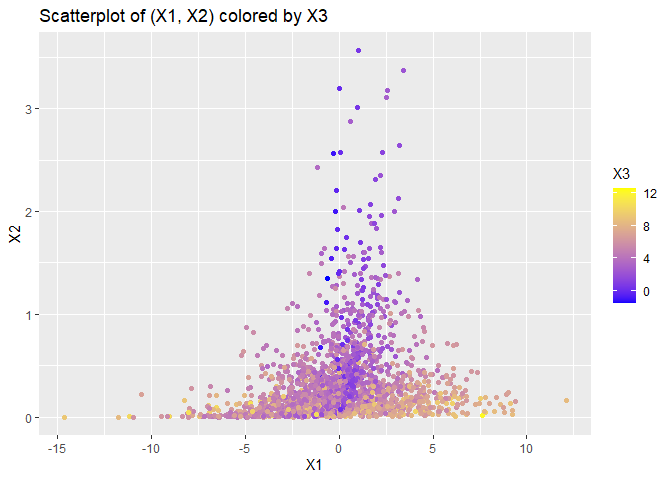We see that the blue points seem quite dependent whereas the yellow points seem less dependent. Let us confirm this by plotting the two subsets separately.

whichX3Low = which(X3 <= 2)
whichX3High = which(X3 > 8)
ggplot() + geom_point(aes(x=X1[whichX3Low], y=X2[whichX3Low]))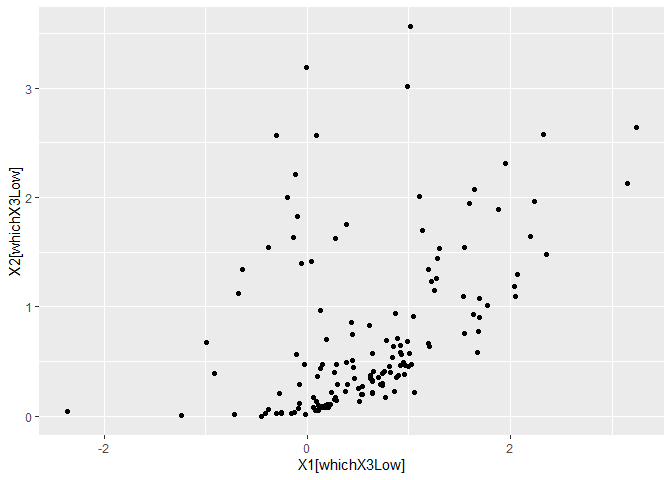ggplot() + geom_point(aes(x=X1[whichX3High], y=X2[whichX3High]))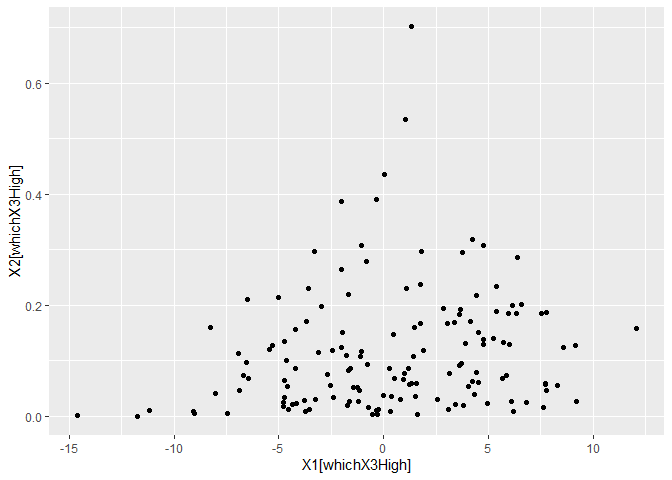It seems that on the first plot, the dependence is quite high between $$X_1$$ and $$X_2$$, while it is much smaller in the second plot. This will be confirmed in the next section.

# Parametric estimation of the conditional copula

In this part, we assume that we know that for each value of the conditioning variable $$X_3$$, the conditional copula of $$X_1$$ and $$X_3$$ belongs to the Clayton family. We can therefore estimate the parameter of the conditional copula by the conditional version of the canonical Maximum Likelihood Estimation. After the estimation, we can then compare the estimated conditional parameters with the true conditional parameter of the Clayton copula.

newX3 = seq(2, 9, by = 0.1)

vecEstimatedThetas = estimateParCondCopula(
observedX1 = X1, observedX2 = X2, observedX3 = X3, newX3 = newX3, family = 3, h = 0.08)

trueCKT12_3 = ckt12_3_fun(newX3)

comparison = data.frame(
x3    = rep(newX3, 2),
param = c(vecEstimatedThetas , BiCopTau2Par(copFamily12_3, trueCKT12_3)),
CKT   = c(BiCopPar2Tau(copFamily12_3, vecEstimatedThetas) , trueCKT12_3),
id    = rep(1:2,each=length(newX3)))

ggplot(data = comparison) +
geom_line(aes(x = x3, y = param, color = as.factor(id), group = as.factor(id))) +
scale_color_discrete(labels = c("Estimated parameter", "True parameter"), name="") +
xlab("Conditioning value x3") +
ylab(expression(paste("Conditional parameter ",theta,"(x3)"))) +
ggtitle(paste0("Comparison between the estimated conditional parameter\n",
"of the Clayton copula and the true conditional parameter\n",
"as functions of the conditioning variable X3"))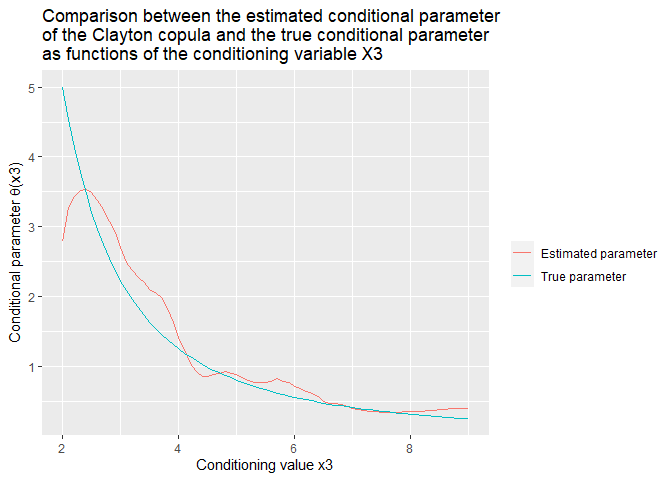Since there exists a bijection between the parameter of the conditional copula and the associated Kendall’s tau, this can also give us an estimator of the conditional Kendall’s tau. In the next graph, we compare this estimator with the true value of the conditional Kendall’s tau.


ggplot(data = comparison, aes(x = x3, y = CKT, color = as.factor(id), group = as.factor(id))) +
geom_line() +
scale_color_discrete(labels = c("Estimated CKT","True CKT"), name="") +
xlab("Conditioning value x3") +
ylab("Conditional Kendall's tau") +
ggtitle(paste0("Comparison between the estimated conditional Kendall's tau\n",
"and the true conditional Kendall's tau\n",
"as functions of the conditioning variable X3"))